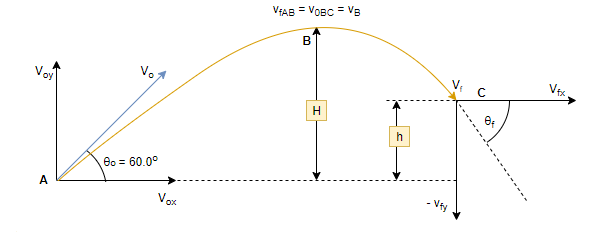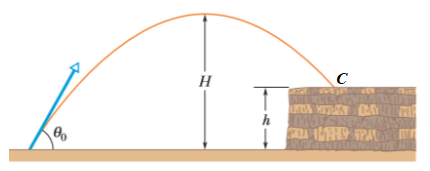# Problem: In the figure, a stone is projected at a cliff of height  h with an initial speed of 42.0 m/s directed at angle θ0 = 60.0° above the horizontal. The stone strikes at C, 5.50 s after launching. Find (a) the height h of the cliff, (b) the speed of the stone just before impact at C, and (c) the maximum height H reached above the ground.

###### FREE Expert Solution

In this problem, we’re asked to calculate the height of the cliff the stone lands on, the final speed of the stone, and the maximum height the stone reaches during its motion.

This is a projectile motion problem with a positive launch angle. For projectile motion problems, we follow the following simple steps:

1. Draw diagram, axes.
2. Develop equations to describe the various intervals.
3. Solve each of the target variables.

Step 1: Draw diagram, axes.For projectiles with a positive launch angle, the motion has two parts: AB, from launch to peak, and BC, from peak to when it lands. We use AC for the full motion.

We'll consider the x and y speed components separately. Therefore, to resolve v0 to v0x and v0y, we apply trigonometry

vx = 21.0 m/s

The horizontal speed (x-component of v0) is constant since there is no horizontal acceleration.

v0y = 36.4 m/s

The acceleration of the stone before reaching maximum height causes a decrease in vertical speed, vy while the acceleration after the maximum height increases the vertical speed, vy, downwards.

We will also use a standard coordinate system where upward motion is positive y and downward motion is negative y.  Also, the stone moves to the right in the positive x-direction.

97% (393 ratings)###### Problem Details

In the figure, a stone is projected at a cliff of height  h with an initial speed of 42.0 m/s directed at angle θ0 = 60.0° above the horizontal. The stone strikes at C, 5.50 s after launching. Find

(a) the height h of the cliff,

(b) the speed of the stone just before impact at C, and

(c) the maximum height H reached above the ground.Frequently Asked Questions

What scientific concept do you need to know in order to solve this problem?

Our tutors have indicated that to solve this problem you will need to apply the Positive (Upward) Launch concept. You can view video lessons to learn Positive (Upward) Launch. Or if you need more Positive (Upward) Launch practice, you can also practice Positive (Upward) Launch practice problems.

What professor is this problem relevant for?

Based on our data, we think this problem is relevant for Professor Zamani's class at HCC.

What textbook is this problem found in?

Our data indicates that this problem or a close variation was asked in Fundamentals of Physics - Halliday Calc 10th Edition. You can also practice Fundamentals of Physics - Halliday Calc 10th Edition practice problems.